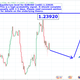Long
134 views
Equilibrium state of a financial instrument/market
All financial instrument(s) or markets have local or global equilibrium states. For example, if S(t) represents the value of a financial asset, S , at time t and the expectation, E(S(t))
, of the stochastic process, S(t), is finite then the financial instrument, S, has a global equilibrium state. There is a number that S(t) will return to, infinitely often. The problem of finding a local equilibrium state is more difficult and we have found a way around this difficult problem. Well, the price of a financial asset depends on momentum and no one can really control when
people buy or sell a particular asset. However,
we can estimate, very well, where local
equilibrium states of a number of assets are.
Once we determine that is the closest local
equilibrium state of a given financial asset
currently, it means that within some finite time
period, which can be estimated quite well, the
the state , all others things remaining fairly
normal.
For example, if EURUSD -0.06% is currently at 1.2134 and
1.2000 is a local recurrent state for EURUSD -0.06% then,
usually within a week, EURUSD -0.06% is expected to